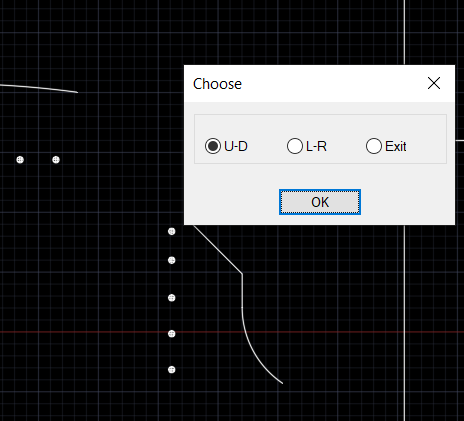# LISP move block (vertical or horizontal) to selected polyline

## Recommended PostsI'm looking for LISP which will help me to move blocks to intersect selected polyline. The problem is:

-I have group of blocks in some  spacing horizontally or vertically

-I have polyline above or below, left or right from this blocks. The polyline consist of few lines in different angles or arc sometimes

-now I'm moving blocks one by one and moving it to intersect polyline

It is possible to make LISP which will do intersecition? For example I,m selecting group of blocks, after that polyline, after that say: X,Y, (maybe up,down,left,right) and now LISP is moving block to intersect polyline?

If it is possible even to do lisp which will make it only UP it will be great. I can always rotate and move polyline and group of blocks. I have hundreds of blocks to move like this...

I've gave attachment what I need.

##### Share on other sitesHere is for UP/DOWN, but I am sure you can figure it also for LEFT/RIGHT...

```(defun c:moveblksup ( / c ss i b p q )

(while
(or
(not (setq c (car (entsel "\nPick curve, placed up or down..."))))
(if c
(vl-catch-all-error-p (vl-catch-all-apply 'vlax-curve-getstartpoint (list c)))
)
)
(prompt "\nMissed or picked entity not curve...")
)
(while
(or
(prompt "\nSelect blocks you want to move up/down until intersecting with previously picked curve...")
(not (setq ss (ssget "_:L" '((0 . "INSERT")))))
)
(prompt "\nEmpty sel.set...")
)
(repeat (setq i (sslength ss))
(setq b (ssname ss (setq i (1- i))))
(setq p (cdr (assoc 10 (entget b))))
(setq q (vlax-curve-getclosestpointtoprojection c p '(0.0 1.0 0.0)))
(if q
(vla-move (vlax-ename->vla-object b) (vlax-3d-point p) (vlax-3d-point q))
)
)
(princ)
)```

##### Share on other sitesu can use array command.

##### Share on other sitesThank You so much @marko_ribar! That exacly what i want to haveChanged it do left and rightmaybe someone will need it

```(defun c:moveblklr ( / c ss i b p q )

(while
(or
(not (setq c (car (entsel "\nPick curve, placed left or right..."))))
(if c
(vl-catch-all-error-p (vl-catch-all-apply 'vlax-curve-getstartpoint (list c)))
)
)
(prompt "\nMissed or picked entity not curve...")
)
(while
(or
(prompt "\nSelect blocks you want to move right/left until intersecting with previously picked curve...")
(not (setq ss (ssget "_:L" '((0 . "INSERT")))))
)
(prompt "\nEmpty sel.set...")
)
(repeat (setq i (sslength ss))
(setq b (ssname ss (setq i (1- i))))
(setq p (cdr (assoc 10 (entget b))))
(setq q (vlax-curve-getclosestpointtoprojection c p '(1.0 0.0 0.0)))
(if q
(vla-move (vlax-ename->vla-object b) (vlax-3d-point p) (vlax-3d-point q))
)
)
(princ)
)```

4 hours ago, Ish said:

u can use array command.

First of all I hate arrays. Groups are better to use for me. Second, maybe I'm don't know this option well, but propably i can't get what i want with array. Last one, it is like that i have blocks in some spacing in few views. In one they are visible as in line in second it must be "attached" to polyline, so for me is better to use group and LISP above. I'm sure that the spacing are the same.

Edited by zwonko

##### Share on other sitesMaybe wrap something like this into the while then run the correct axis option could use initget.```
(defun c:moveblk ( / c ss i b p q )
(while
(or
(not (setq c (car (entsel "\nPick curve"))))
(if c
(vl-catch-all-error-p (vl-catch-all-apply 'vlax-curve-getstartpoint (list c)))
)
)
(prompt "\nMissed or picked entity not curve...")
)
(while
(or
(prompt "\nSelect blocks you want to move  intersecting with previously picked curve...")
(not (setq ss (ssget "_:L" '((0 . "INSERT")))))
)
(prompt "\nEmpty sel.set...")
)
(if (= but nil)(setq but 1))
;(setq ans (ah:butts but "h"  '("Choose" "U-D" "L-R" )))
(setq ans (ah:butts but "v"  '("Choose" "U-D" "L-R" )))
(repeat (setq i (sslength ss))
(setq b (ssname ss (setq i (1- i))))
(setq p (cdr (assoc 10 (entget b))))
(cond
( (= ans "L-R") (setq q (vlax-curve-getclosestpointtoprojection c p '(1.0 0.0 0.0))))
( (= ans "U-D") (setq q (vlax-curve-getclosestpointtoprojection c p '(0.0 1.0 0.0))))
)
(if q
(vla-move (vlax-ename->vla-object b) (vlax-3d-point p) (vlax-3d-point q))
)
)
(princ)
)

(c:moveblk)

```

Edited by BIGAL

##### Share on other sitesThat one is very nicelike I wan't it to bethanks @BIGAL

##### Share on other sitesThanks to Marko for the initial code I just tweaked it a bit.

## Join the conversation

You can post now and register later. If you have an account, sign in now to post with your account.
Note: Your post will require moderator approval before it will be visible.×   Pasted as rich text.   Paste as plain text instead

Only 75 emoji are allowed.